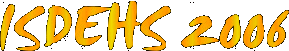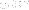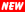International Symposium on
Drylands Ecology and Human Security© 2006 NDRD        Imprint        DisclaimerAssessing the Impact on Groundwater of Solute Transportin Contaminated Soils Using Numerical and Analytical Models Alaa El-Sadek National Water Research Center, Shoubra El-Kheima, Egypte-mail: alaa_elsadek@yahoo.com Abstract Expansion of human activities causes dispersion of pollutants in the subsurface environment. The fate and movement of dissolved substances in soils and groundwater has generated considerable interest out of concern for the quality of the subsurface environment. Many analytical solutions for partial differential equations exist in soil science. Numerical solutions are more general, and often more difficult to verify. Because of the difficulty in obtaining analytical solutions, numerical solutions are using the mathematical model error has to be kept as small as possible. In order to determine the model error, the examination of numerical methods through an analysis of their ability comparing with analytical methods is strongly recommended. The objective of the study is to compare between numerical and analytical solution models for solute transport equation. In this study, the numerical solution calculated with the WAVE-model was compared with the analytical solution calculated with CXTFTT-model. The study scenarios were built up with the combinations of compartment depth, applied flux at the top and soil dispersivity under steady-state conditions. The simulations depend on 27 solute infiltration scenarios. The solute concentrations were calculated with the WAVE-model and the CXTFIT-model for each scenario. The WAVE-model error was evaluated with three methods: absolute average maximum error, relative average maximum error and relative average area error. The study implied that, the WAVE-model error was increasing with the increasing of the compartment depth; the WAVE-model error was decreasing with the increasing of the soil dispersivity; and the WAVE-model error was decreasing with the increasing of the flux. The study recommended using compartment depth as thin as possible to minimize the WAVE-model error. Furthermore, it will be more useful to use several numerical solution models, such as HYDRUS-2D model, to evaluate and examine the WAVE-model. Keywords: numerical solutions; analytical solutions; simulations; steady-state conditions; solute infiltration scenarios